• 这个算法思想就是随机梯度上升算法，他通过随机取数据集中的部分数据，来代表整体数据集，从而实现对数据样本集的缩小，达到减少计算量，降低算法时间复杂度的目的。 3.1. 算法代码 def randGradientAscent ( ...
1. 引言
上一篇日志中，我们最终推导出了计算最优系数的公式。 Logistic 回归数学公式推导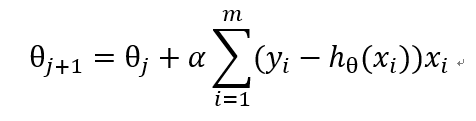本文，我们就利用上一篇文章中计算出的公式来实现模型的训练和数据的分类。
2. 通过 python 实现 logistic 算法
有了上一篇日志中的公式，《机器学习实战》中的代码就非常容易理解了：
# -*- coding:UTF-8 -*-
# {{{
import matplotlib.pyplot as plt
import numpy as np

"""
生成数据矩阵与标签矩阵

:return: 数据矩阵与标签矩阵
"""
dataMat = []
labelMat = []
fr = open('test_dataset.txt')
lineArr = line.strip().split()
""" 每行数据钱添加特征 x0 """
dataMat.append([1.0, float(lineArr), float(lineArr)])
labelMat.append(int(lineArr))
fr.close()
return dataMat, labelMat

def sigmoid(inX):
return 1.0 / (1 + np.exp(-inX))

"""
梯度上升算法

:param dataMatIn: 数据矩阵
:param classLabels: 标签矩阵
:return: 最有参数数组
"""
dataMatrix = np.mat(dataMatIn)
""" 转置 """
labelMat = np.mat(classLabels).transpose()

""" 获取矩阵行数 m 和列数 n """
m, n = np.shape(dataMatrix)
""" 迭代步长 """
alpha = 0.001
""" 迭代次数 """
maxCycles = 500
weights = np.ones((n, 1))
for k in range(maxCycles):
""" 带入数学公式求解 """
h = sigmoid(dataMatrix * weights)
weights = weights + alpha * dataMatrix.transpose() * (labelMat - h)
""" 转换为 array """
return weights.getA()

def plotBestFit(weights):
"""
绘制数据集与结果分类线

:param weights: 权重参数数组
:return:
"""
dataArr = np.array(dataMat)

""" 数据个数 """
n = np.shape(dataMat)

""" 测试数据两组分类结果集 """
xcord1 = []
ycord1 = []
xcord2 = []
ycord2 = []

""" 对数据进行分类 """
for i in range(n):
if int(labelMat[i]) == 1:
xcord1.append(dataArr[i, 1])
ycord1.append(dataArr[i, 2])
else:
xcord2.append(dataArr[i, 1])
ycord2.append(dataArr[i, 2])

""" 绘图 """
fig = plt.figure()
""" 分割画布为一整份，占据其中一整份 """
""" 离散绘制分类结果为 1 的样本 """
ax.scatter(xcord1, ycord1, s=20, c='red', marker='s', alpha=.5)
""" 离散绘制分类结果为 0 的样本 """
ax.scatter(xcord2, ycord2, s=20, c='green', alpha=.5)
x1 = np.arange(-3.0, 3.0, 0.1)
x2 = (-weights - weights * x1) / weights
ax.plot(x1, x2)
plt.title('BestFit')
plt.xlabel('X1')
plt.ylabel('X2')
plt.show()

if __name__ == '__main__':
plotBestFit(weights)
# }}}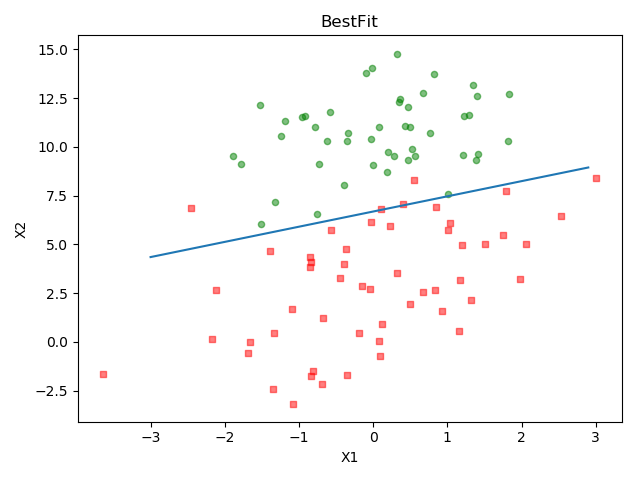训练样本数据见本文的附录。
3. 随机梯度上升算法
当数据量达到上亿或更多数据以后，梯度上升算法中的矩阵乘法等操作显然耗时将上升到非常高的程度，那么，我们是否可以不用整个数据集作为样本来计算其权重参数而是只使用其中的一部分数据来训练呢？ 这个算法思想就是随机梯度上升算法，他通过随机取数据集中的部分数据，来代表整体数据集，从而实现对数据样本集的缩小，达到减少计算量，降低算法时间复杂度的目的。
3.1. 算法代码
def randGradientAscent(dataMatrix, classLabels, numIter=20):
"""
随机梯度上升算法

:param dataMatrix: 数据矩阵
:param classLabels: 标签矩阵
:param numIter: 外循环次数
:return: 权重数组与回归系数矩阵
"""
time0 = time.time()
m,n = np.shape(dataMatrix)
weights = np.ones(n)
weights_array = np.array([])
innerIterNum = int(m/100)
for j in range(numIter):
dataIndex = list(range(m))
for i in range(innerIterNum):
alpha = 4/(1.0 + j + i) + 0.01  # 降低alpha的大小，每次减小1/(j+i)
""" 随机选取样本 """
randIndex = int(random.uniform(0,len(dataIndex)))
chose = dataIndex[randIndex]
h = sigmoid(sum(dataMatrix[chose]*weights))
error = classLabels[chose] - h
weights = weights + alpha * error * dataMatrix[chose]
weights_array = np.append(weights_array,weights,axis=0)
del(dataIndex[randIndex])
weights_array = weights_array.reshape(numIter*innerIterNum, n)
print("随机梯度算法耗时：", time.time() - time0)
return weights, weights_array

上述代码与梯度上升算法相比，主要有以下改进：
将原有的迭代 + 矩阵操作替换成内外层双层循环实现，内循环只随机选取原数据集的 1/100 规模，从而实现计算量的缩减alpha 动态调整，随着内循环的进行，逐步缩小，从而对获取更准确的最优值与运行时间二者的优化
4. 随机梯度上升算法与梯度上升算法效果对比
下面代码对比了梯度上升算法与随机梯度上升算法的效果。
# -*- coding:UTF-8 -*-
# {{{
import time

from matplotlib.font_manager import FontProperties
import matplotlib.pyplot as plt
import numpy as np
import random

"""
加载数据

:return: 数据集，标签集
"""
dataMat = []
labelMat = []
fr = open('test_dataset.txt')
for i in range(100):
lineArr = line.strip().split()
dataMat.append([1.0, float(lineArr), float(lineArr)])
labelMat.append(int(lineArr))
fr.close()
return dataMat, labelMat

def sigmoid(inX):
return 1.0 / (1 + np.exp(-inX))

def gradAscent(dataMatIn, classLabels, maxCycles = 300):
"""
梯度上升算法

:param dataMatIn: 数据矩阵
:param classLabels: 数据集
:param maxCycles: 迭代次数
:return: 权重数组与回归系数矩阵
"""
time0 = time.time()
dataMatrix = np.mat(dataMatIn)
labelMat = np.mat(classLabels).transpose()
m, n = np.shape(dataMatrix)
alpha = 0.0001
weights = np.ones((n,1))
weights_array = np.array([])
for k in range(maxCycles):
h = sigmoid(dataMatrix * weights)
error = labelMat - h
weights = weights + alpha * dataMatrix.transpose() * error
weights_array = np.append(weights_array,weights)
weights_array = weights_array.reshape(maxCycles,n)
print("梯度上升算法耗时：", time.time() - time0)
return weights.getA(), weights_array

"""
随机梯度上升算法

:param dataMatrix: 数据矩阵
:param classLabels: 标签矩阵
:param numIter: 外循环次数
:return: 权重数组与回归系数矩阵
"""
time0 = time.time()
m,n = np.shape(dataMatrix)
weights = np.ones(n)
weights_array = np.array([])
innerIterNum = int(m/100)
for j in range(numIter):
dataIndex = list(range(m))
for i in range(innerIterNum):
alpha = 4/(1.0 + j + i) + 0.01  #降低alpha的大小，每次减小1/(j+i)
""" 随机选取样本 """
randIndex = int(random.uniform(0,len(dataIndex)))
chose = dataIndex[randIndex]
h = sigmoid(sum(dataMatrix[chose]*weights))
error = classLabels[chose] - h
weights = weights + alpha * error * dataMatrix[chose]
weights_array = np.append(weights_array,weights,axis=0)
del(dataIndex[randIndex])
weights_array = weights_array.reshape(numIter*innerIterNum, n)
print("随机梯度算法耗时：", time.time() - time0)
return weights, weights_array

"""
绘制回归系数与迭代次数的关系

:return:
"""

font = FontProperties(fname=r"c:\windows\fonts\simsun.ttc", size=14)
"""
将fig画布分隔成1行1列,不共享x轴和y轴,fig画布的大小为 (13,8)，分为六个区域
"""
fig, axs = plt.subplots(nrows=3, ncols=2, sharex='none', sharey='none', figsize=(20,10))

axs0_title_text = axs.set_title(u'改进的随机梯度上升算法：回归系数与迭代次数关系',FontProperties=font)
axs0_ylabel_text = axs.set_ylabel(u'W0',FontProperties=font)
plt.setp(axs0_title_text, size=20, weight='bold', color='black')
plt.setp(axs0_ylabel_text, size=20, weight='bold', color='black')

axs1_ylabel_text = axs.set_ylabel(u'W1',FontProperties=font)
plt.setp(axs1_ylabel_text, size=20, weight='bold', color='black')

axs2_xlabel_text = axs.set_xlabel(u'迭代次数',FontProperties=font)
axs2_ylabel_text = axs.set_ylabel(u'W1',FontProperties=font)
plt.setp(axs2_xlabel_text, size=20, weight='bold', color='black')
plt.setp(axs2_ylabel_text, size=20, weight='bold', color='black')

axs0_title_text = axs.set_title(u'梯度上升算法：回归系数与迭代次数关系',FontProperties=font)
axs0_ylabel_text = axs.set_ylabel(u'W0',FontProperties=font)
plt.setp(axs0_title_text, size=20, weight='bold', color='black')
plt.setp(axs0_ylabel_text, size=20, weight='bold', color='black')

axs1_ylabel_text = axs.set_ylabel(u'W1',FontProperties=font)
plt.setp(axs1_ylabel_text, size=20, weight='bold', color='black')

axs2_xlabel_text = axs.set_xlabel(u'迭代次数',FontProperties=font)
axs2_ylabel_text = axs.set_ylabel(u'W1',FontProperties=font)
plt.setp(axs2_xlabel_text, size=20, weight='bold', color='black')
plt.setp(axs2_ylabel_text, size=20, weight='bold', color='black')

plt.show()

if __name__ == '__main__':
plotWeights(weights_array1, weights_array2)
# }}}

首先将数据集通过复制 100 次，从而实现将原有训练数据集从 100 个变为 10000 个的效果。 然后，我们画出了迭代过程中的权重变化曲线。
4.1. 输出结果
输出了：
随机梯度算法耗时： 0.03397965431213379
梯度上升算法耗时： 0.11360883712768555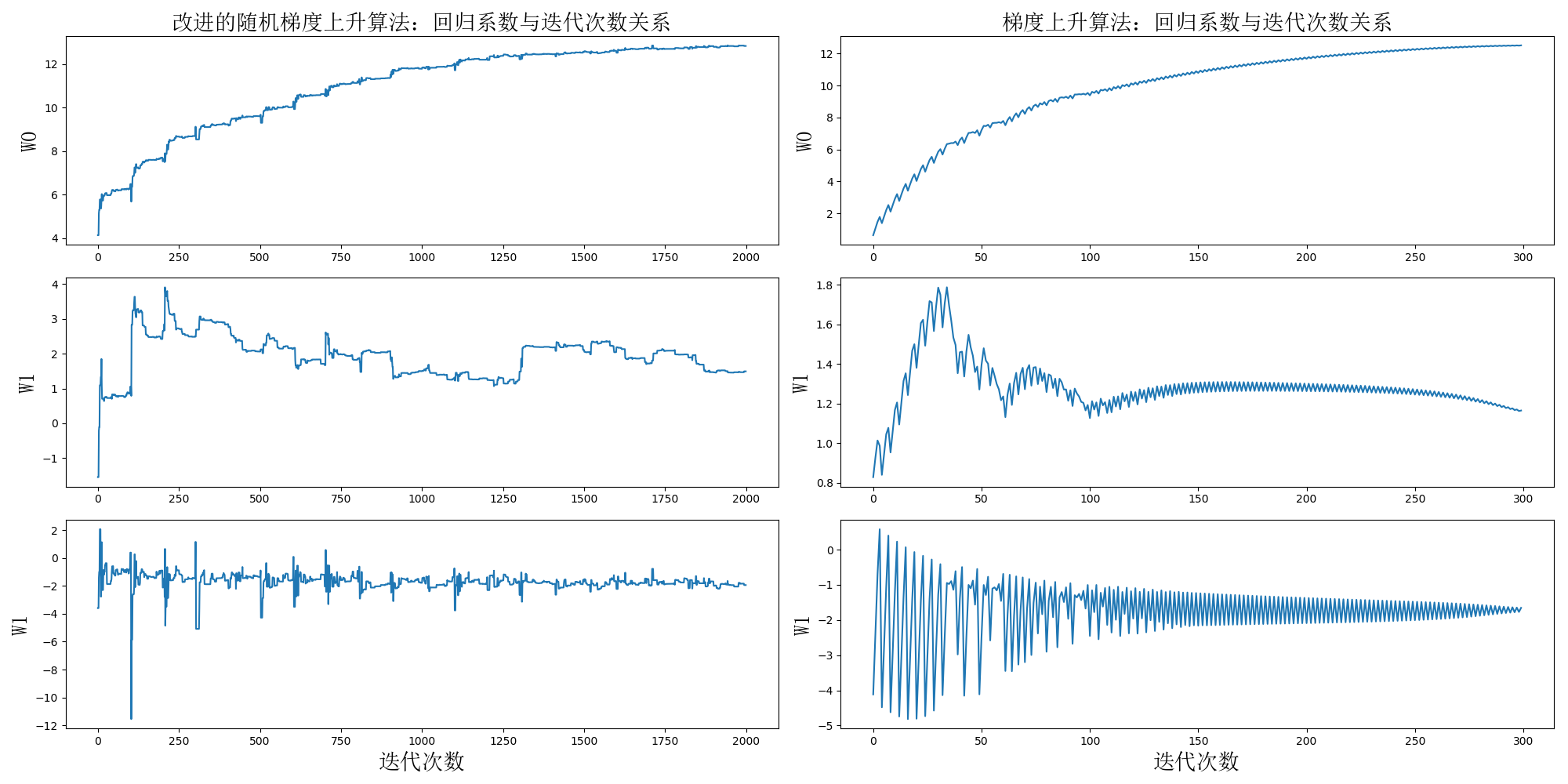4.2. 结果分析
可以看到，两个算法都刚好在我们迭代结束时趋于平衡，这是博主为了对比两个算法运行的时间对迭代次数进行多次调整的结果。 结果已经非常明显，虽然从波动范围来看，随机梯度上升算法在迭代过程中更加不稳定，但随机梯度上升算法的收敛时间仅仅是梯度上升算法的30%，时间大为缩短，如果数据规模进一步上升，则差距将会更加明显。 而从结果看，两个算法的最终收敛位置是非常接近的，但是，从原理上来说，随机梯度算法效果确实可能逊于梯度上升算法，但这仍然取决于步进系数、内外层循环次数以及随机样本选取数量的选择。
5. 《机器学习实战》随机梯度上升算法讲解中的错误
几天前，阅读《机器学习实战》时，对于作者所写的代码例子，有很多疑问，经过几天的研究，确认是某种原因导致的谬误，最终有了上文中博主自己改进过的代码，实现了文中的算法思想。 这里详细来说一下书中源码和结论中存在的纰漏。 下面代码是书中的源代码：
def stocGradAscent1(dataMatrix, classLabels, numIter=150):
m,n = shape(dataMatrix)
weights = ones(n)   #initialize to all ones
for j in range(numIter):
dataIndex = range(m)
for i in range(m):
alpha = 4/(1.0+j+i)+0.0001    #apha decreases with iteration, does not
randIndex = int(random.uniform(0, len(dataIndex)))#go to 0 because of the constant
h = sigmoid(sum(dataMatrix[randIndex]*weights))
error = classLabels[randIndex] - h
weights = weights + alpha * error * dataMatrix[randIndex]
del(dataIndex[randIndex])
return weights

这段代码存在下面的几个问题，尽信书不如无书，网上很多笔记和博客都是照搬书上的代码和结论，而没有进行自己的思考，这样是不可取的。
5.1. 随机样本选择时下标问题
代码中，randIndex 是从 0 到 dataIndex 长度 - 1的随机数，假设训练数据规模为 100。 那么首次迭代中，dataIndex 是从 0 到 99 的随机数，假设 dataIndex 取到的是 0，那么本次迭代的训练数据是第 0 条数据，然后，首次迭代的最后，删除了 dataIndex 的第 0 个元素。 第二次迭代中，dataIndex 是从 0 到 98 的随机数，假设 dataIndex 取到的是 0，那么本次迭代的训练数据是第 0 条数据，然后，第二次迭代的最后，删除了 dataIndex 的第 0 个元素。 。。。 如果刚好我们的 dataIndex 每次都取到 0，那么最终的训练结果将毫无意义。
首先，即使这段代码是正确的，del(dataIndex[randIndex]) 的意义是什么呢？dataIndex 列表存在的价值又是什么呢？为什么不直接用一个数字代表规模来进行每次内迭代中的自减操作呢？ 所以代码原意是：
randIndex = dataIndex[int(random.uniform(0, len(dataIndex)))]

这样才是正确的。
5.2. 为什么内迭代 m 次
内迭代 m 次的结果是什么呢？是将数据集按行打乱顺序。 从矩阵运算的规则来看，将矩阵运算变成每一行拆分运算，其结果是完全等价的，而 python 算法库中，矩阵运算是通过 CPython 转化为 C 语言进行的，其运算速度是要明显优于我们自己拆分运算的。 对于整个算法来说，打乱样本数据的排列是完全没有任何意义的。 而事实上，在《机器学习实战》的文中，也提到，随机梯度上升算法是通过选取样本数据集的子集进行计算来实现效率的提升的，而这个思想并不是代码中所反映出的思想。
5.3. 随机梯度算法收敛速度更快
作者在运行结果中，对比了随机梯度上升算法与梯度上升算法达到收敛时的迭代次数。 首先，迭代内部算法不同，单纯比较迭代次数有什么意义呢？ 而作者竟然将上述代码的外循环次数与梯度上升算法的迭代次数进行比较，这样的比较结果是在愚弄读者吧？
5.4. 随机选取机制可以减小波动？
书中对比随机梯度算法与梯度上升算法的权重迭代曲线，得出结论：这里的系数没有像之前那样出现周期性波动，这归功于样本随机选择机制。 无论是算法原理还是从作者贴出的图来看都不能得到这样的结论。
6. 附录
6.1. 训练样本数据
-0.017612       14.053064       0
-1.395634       4.662541        1
-0.752157       6.538620        0
-1.322371       7.152853        0
0.423363        11.054677       0
0.406704        7.067335        1
0.667394        12.741452       0
-2.460150       6.866805        1
0.569411        9.548755        0
-0.026632       10.427743       0
0.850433        6.920334        1
1.347183        13.175500       0
1.176813        3.167020        1
-1.781871       9.097953        0
-0.566606       5.749003        1
0.931635        1.589505        1
-0.024205       6.151823        1
-0.036453       2.690988        1
-0.196949       0.444165        1
1.014459        5.754399        1
1.985298        3.230619        1
-1.693453       -0.557540       1
-0.576525       11.778922       0
-0.346811       -1.678730       1
-2.124484       2.672471        1
1.217916        9.597015        0
-0.733928       9.098687        0
-3.642001       -1.618087       1
0.315985        3.523953        1
1.416614        9.619232        0
-0.386323       3.989286        1
0.556921        8.294984        1
1.224863        11.587360       0
-1.347803       -2.406051       1
1.196604        4.951851        1
0.275221        9.543647        0
0.470575        9.332488        0
-1.889567       9.542662        0
-1.527893       12.150579       0
-1.185247       11.309318       0
-0.445678       3.297303        1
1.042222        6.105155        1
-0.618787       10.320986       0
1.152083        0.548467        1
0.828534        2.676045        1
-1.237728       10.549033       0
-0.683565       -2.166125       1
0.229456        5.921938        1
-0.959885       11.555336       0
0.492911        10.993324       0
0.184992        8.721488        0
-0.355715       10.325976       0
-0.397822       8.058397        0
0.824839        13.730343       0
1.507278        5.027866        1
0.099671        6.835839        1
-0.344008       10.717485       0
1.785928        7.718645        1
-0.918801       11.560217       0
-0.364009       4.747300        1
-0.841722       4.119083        1
0.490426        1.960539        1
-0.007194       9.075792        0
0.356107        12.447863       0
0.342578        12.281162       0
-0.810823       -1.466018       1
2.530777        6.476801        1
1.296683        11.607559       0
0.475487        12.040035       0
-0.783277       11.009725       0
0.074798        11.023650       0
-1.337472       0.468339        1
-0.102781       13.763651       0
-0.147324       2.874846        1
0.518389        9.887035        0
1.015399        7.571882        0
-1.658086       -0.027255       1
1.319944        2.171228        1
2.056216        5.019981        1
-0.851633       4.375691        1
-1.510047       6.061992        0
-1.076637       -3.181888       1
1.821096        10.283990       0
3.010150        8.401766        1
-1.099458       1.688274        1
-0.834872       -1.733869       1
-0.846637       3.849075        1
1.400102        12.628781       0
1.752842        5.468166        1
0.078557        0.059736        1
0.089392        -0.715300       1
1.825662        12.693808       0
0.197445        9.744638        0
0.126117        0.922311        1
-0.679797       1.220530        1
0.677983        2.556666        1
0.761349        10.693862       0
-2.168791       0.143632        1
1.388610        9.341997        0
0.317029        14.739025       0

欢迎关注微信公众号参考资料
Peter Harrington 《机器学习实战》。 李航 《统计学习方法》。 https://en.wikipedia.org/wiki/Maximum_likelihood_estimation。 https://en.wikipedia.org/wiki/Gradient_descent。 https://blog.csdn.net/c406495762/article/details/77851973。 https://blog.csdn.net/charlielincy/article/details/71082147。
展开全文机器学习 logistic回归 梯度上升 机器学习实战
• 并通过引入Sigmoid函数和梯度公式成功推导出了梯度上升和梯度下降公式，上文分类实例是依据全批量提升上升法，而本文会介绍全批量梯度上升的一种优化算法——随机梯度上升，如果还未懂得逻辑回归思想和推理公式的...
前言概述
上一篇文章对逻辑回归的原理和基本思想做了一些简要介绍，并通过引入Sigmoid函数和梯度公式成功推导出了梯度上升和梯度下降公式，上文分类实例是依据全批量提升上升法，而本文会介绍全批量梯度上升的一种优化算法——随机梯度上升，如果还未懂得逻辑回归思想和推理公式的原理，还请观看上一篇文章：机器学习笔记(七)——初识逻辑回归、不同方法推导梯度公式。
随机梯度上升
区别对比
在讲解全批量梯度上升和随机梯度上升的区别之前，先看一下二者的公式之间的对比，有助于之后的理解。 全批量梯度上升法：随机梯度上升法：全批量梯度上升公式我们已经很熟悉，在上一篇文章有介绍；其实随机梯度上升与全批量的公式十分相似，原理也是大致相同的，不同点体现在何处呢？全批量在每次更新回归系数时都需要遍历整个数据集，这种方法在处理小数据集时尚可，但如果有数十亿样本和成千上万的特征，那么该方法的计算复杂度太高。而随机梯度上升是一次仅用一个样本点来更新回归系数，这样做大大减小了计算复杂度，并且提高了函数的收敛速度。
更改算法
随机梯度上升算法伪代码如下
所有回归系数初始化为1
对数据集中每个样本
计算该样本的梯度
返回回归系数值

因为两个公式大体上一致，所以随机梯度上升法的代码只会稍微有些出入。
def stocGradAscent1(dataMatrix, classLabels):
#将列表转化为array格式
dataMatrix = np.array(dataMatrix)
# 获取dataMatrix的行、列数
m,n = np.shape(dataMatrix)
# 初始化回归系数和步长
weights = np.ones(n)
alpha = 0.01
for i in range(m):
# 遍历样本，每次选出一个，计算h
h = sigmoid(sum(dataMatrix[i]*weights))
# 计算误差
error = classLabels[i] - h
# 更新回归系数
weights = weights + alpha * error * dataMatrix[i]
return weights

可以看到两种算法有些区别：第一，随机梯度上升的变量h和误差error都是数值，而全批量中二者都是向量格式；第二，随机梯度没有矩阵运算，所有变量的数据类型都为Numpy数组。
执行上述代码可以得到一条新的最佳拟合直线图，如下：可以看到，这条新的最佳拟合直线只分对了二分之一的样本。明明是对算法进行调优，可是准确率怎么越调越低呢？原因是全批量梯度上升法是在整个数据集上迭代了500次才得到的，迭代次数要远大于随机梯度方法，而判断一个算法优劣的可靠方法是看它是否收敛，也就是参数是否达到了稳定值，是否还会不断变化。
下图为两种方法迭代次数与回归系数之间的关系：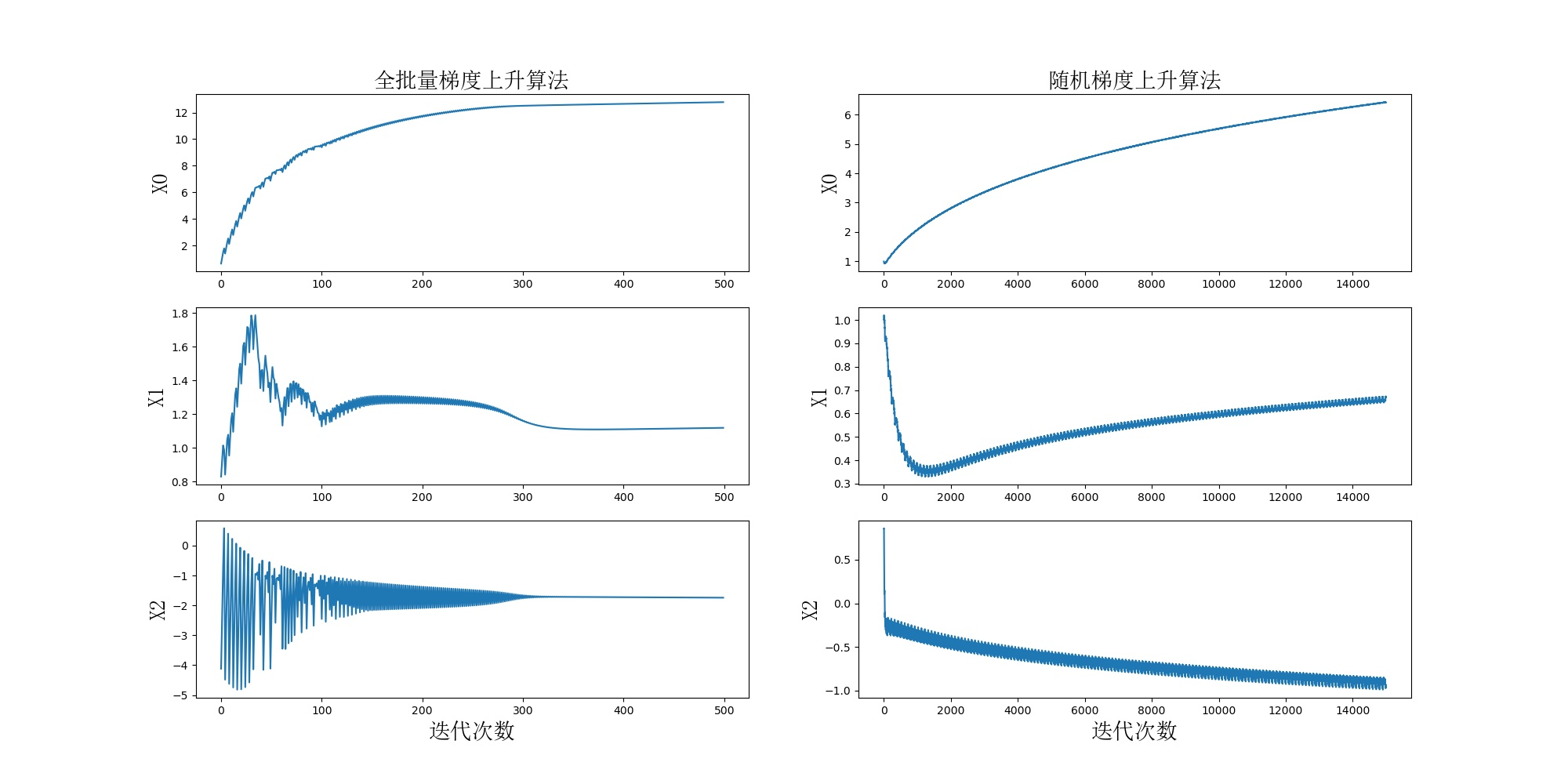可以看到全批量梯度三个回归图像与随机梯度相比波动幅度都比较大，用最明显的回归系数X2作比较，前者在下标为300时收敛完成，而后者在下标14000时曲线才近乎平稳；但这里需要注意的是，全批量梯度每次迭代都是利用整个数据集，所以该方法收敛完成时的准确迭代次数应该是30000次，比随机梯度的迭代次数要多的多。
随机梯度方法在大的波动之后，还有很多小的周期波动，产生这种现象的原因是存在一些不能正确分类的样本点，在每次迭代时会引发系数的剧烈改变。我们希望算法可以避免波动问题，从而收敛到某个值，并且加快收敛速度。
算法调优
所以对随机梯度上升算法做以下改进：
def stocGradAscent2(dataMatrix, classLabels, numIter=150):
#将列表转化为array格式
dataMatrix = np.array(dataMatrix)
# 获取dataMatrix的行、列数
m,n = np.shape(dataMatrix)
# 初始化回归系数和步长
weights = np.ones(n)
for j in range(numIter):
dataIndex = list(range(m))
for i in range(m):
# 逐渐减小alpha，每次减小量为1.0/(j+i)
1、alpha = 4/(1.0+j+i)+0.01
# 随机选取样本
2、randIndex = int(random.uniform(0,len(dataIndex)))
# 随机选取的一个样本，计算h
h = sigmoid(sum(dataMatrix[randIndex]*weights))
# 计算误差
error = classLabels[randIndex] - h
# 更新回归系数
weights = weights + alpha * error * dataMatrix[randIndex]
# 删除已经使用过的样本
del(dataIndex[randIndex])
return weights

第一处改进目的在于每一次迭代都会调整步长alpha的值，alpha每次迭代都会减小1/(j+i),其中j是迭代次数，i是样本点的下标。虽然alpha会随着迭代次数不断减小，但永远不会减小到0，其中还存在一个常数项，这是因为在多次迭代之后alpha的值近乎为0，这样新数据对于回归系数的更新几乎没有作用。
这里再利用图片再讲解一下为什么要这样优化alpha。上图是一个二次函数的图像，在最开始时梯度较大，步长alpha可以比较大，但梯度是呈现逐渐减小趋势的，这时离最优值也越来越近，步长alpha也要随之减小。如果下降速率很大，在接近最优点时，梯度乘以了一个数值比较大的alpha，就会出现下图这类情况。例如从点1直接跳到了点2，开始震荡，上述迭代次数与回归系数关系图中的较大震荡产生的原因，而上述对步长alpha的优化即可避免这类情况，虽然举例用的是梯度下降，但梯度上升和梯度下降的原理是一致的。
第二处改进的目的是减少随机梯度关系图中周期性的波动，这里通过随机选取抽样样本更新回归系数，每次随机从列表中选取一个值，然后从列表中删掉该值，再进行下一次迭代，与决策树选取信息增益的方式类似。
下图给出了改进后的随机梯度上升法迭代次数与回归系数的关系图。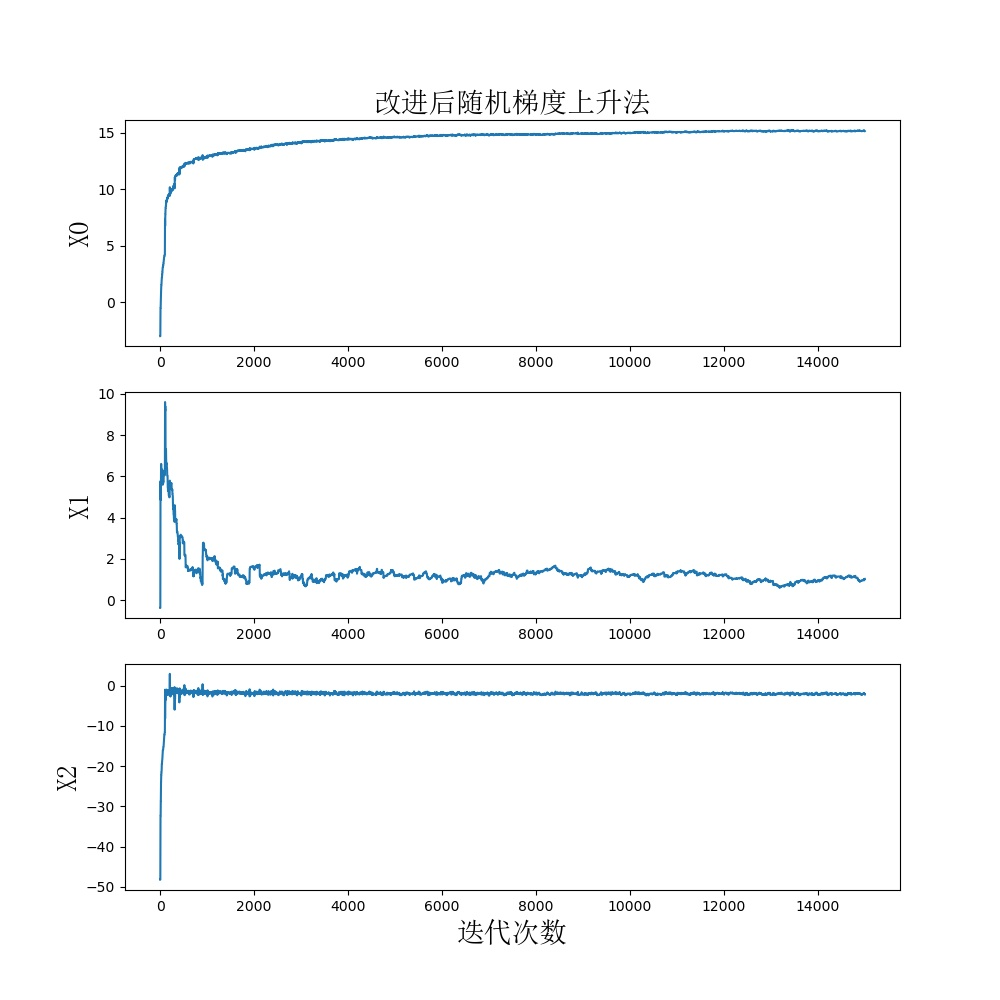可以看出这种方法三个回归系数图像比固定步长alpha的方法收敛速度都要快，并且没有了周期性的波动，为了更直观地看到改进算法的效果，下图给出了利用改进后的算法绘制出的最佳拟合直线图。最终随机梯度的分类效果与全批量梯度几乎一样，但是迭代次数却要少很多很多，所以前者很大程度上降低了算法的计算复杂度，减小了程序使用的内存。
总结
文末总结一下全批量梯度下降法、随机梯度下降法、小批量梯度下降法的优缺点即适应场合。
全批量梯度下降法(BGD)：每次更新回归系数所有样本都参与。
优点：分类准确，获取全局最优解缺点：当样本比较多时，训练速度特别慢适用场合：样本较少的数据集
随机梯度下降法(SGD)：每次更新回归系数只有一个样本参与。
优点：训练速度很快缺点：准确率会降低，并不是朝着整体最优方向进行，容易获取到局部最优解适用场合：样本非常多的数据集
小批量梯度下降法(MBGD)：每次更新回归系数有一部分样本参与。这种方法兼顾了上述两种方法的优点，同时也减弱了两者的缺点，算是两种前两种算法的一种平衡。如果数据集的样本数不是很极端，最好采用小批量梯度下降法。

关注公众号【喵说Python】后台回复“随机梯度”可获取源码供参考，感谢阅读。

展开全文python 机器学习 逻辑回归 分类算法 人工智能
• 训练算法：随机梯度上升 &gt;&gt;&gt; np.ones(5) array([ 1., 1., 1., 1., 1.]) &gt;&gt;&gt; np.ones((5,), dtype=np.int) array([1, 1, 1, 1, 1]) &gt;&gt;&gt; np....
训练算法：随机梯度上升
>>> np.ones(5)
array([ 1.,  1.,  1.,  1.,  1.])

>>> np.ones((5,), dtype=np.int)
array([1, 1, 1, 1, 1])

>>> np.ones((2, 1))
array([[ 1.],
[ 1.]])

>>> s = (2,2)
>>> np.ones(s)
array([[ 1.,  1.],
[ 1.,  1.]])
随机梯度上升算法
def stocGradAscent0(dataMatrix, classLabels):
m,n = shape(dataMatrix)
alpha = 0.01
weights = ones(n)
for i in range(m):
h = sigmoid(sum(dataMatrix[i]*weights))
error = classLabels[i] - h
weights = weights + alpha * error * dataMatrix[i]
return weights
随机梯度上升算法与梯度上升算法在代码上很相似，但也有一些区别：第一，后者的变量和误差都是向量，而前者则全是数值；第二，前者没有矩阵的转换过程，所有的变量的数据类型都是Numpy数组
>>> import logRegres
<module 'logRegres' from 'E:\\Python\\logRegres.py'>
>>> logRegres.plotBestFit(weights)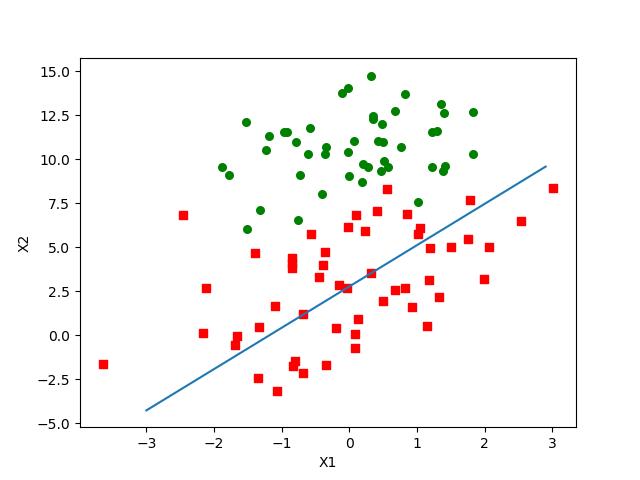可以看到，拟合出来的直线效果还不错，但并不像之前那么完美，这里的分类器错分了三分之一的样本。
改进的随机梯度上升算法  random.uniform(x, y) 方法将随机生成一个实数，它在 [x,y] 范围内。
def stocGradAscent1(dataMatrix, classLabels, numIter=150):
m,n = shape(dataMatrix)
weights = ones(n)
for j in range(numIter):
dataIndex = list(range(m))
for i in range(m):
alpha = 4/(1.0+j+i)+0.01
randIndex = int(random.uniform(0,len(dataIndex)))
h = sigmoid(sum(dataMatrix[randIndex]*weights))
error = classLabels[randIndex] - h
weights = weights + alpha * error * dataMatrix[randIndex]
del(dataIndex[randIndex])
return weights
第一个改进的地方alpha = 4/(1.0+j+i)+0.01  一方面，alpha在每次迭代的时候都会调整，这回缓解数据波动或者高频波动。另外，虽然alpha会随着迭代次数不断减小，但永远不会减小到0，这是因为其中还存在一个常数项。  必须这样做的原因是为了保证在多次迭代之后新数据依然具有一定的影响。如果要处理的问题是动态变化的，那么可以适当加大上述常数项，来确保新的值获得更大的回归系数。  第二个改进的地方 randIndex = int(random.uniform(0,len(dataIndex)))  通过随机选择样本来更新回归系数，这种方法将减少周期性的波动。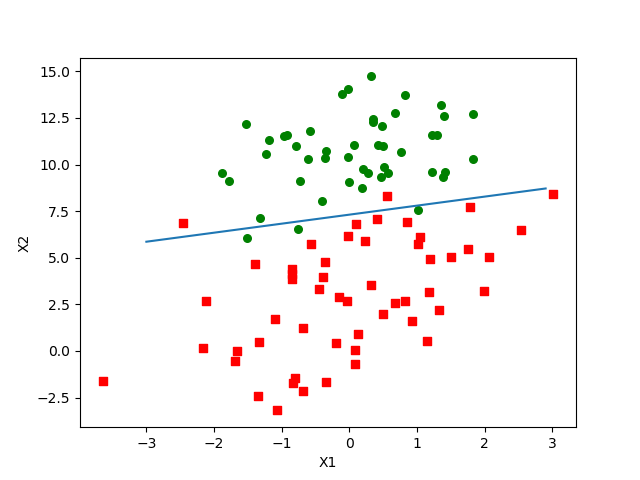展开全文• 前言 前面通过梯度上升法实现求解最佳回归系数，虽然能够达到不错的效果，但是实现过程需要进行大量的计算(dataMatrix*weights进行了300次相乘，实现过程)。所以对该算法进行改进。
前言
前面通过梯度上升法实现求解最佳回归系数，虽然能够达到不错的效果，但是实现过程需要进行大量的计算(dataMatrix*weights进行了300次相乘，实现过程)。所以对该算法进行改进。
随机梯度上升算法
梯度上升算法在每次更新回归系数的时候，都需要遍历整个数据集，所以在处理小的数据集的时候还不错，一旦数据集过大，计算复杂度就太大了。 可以一次只用一个样本点来更新回归系数，这就是随机梯度上升算法。
随机梯度上升
def stoc_grad_ascent(dataSet, labelSet, numIter=150):
m, n = shape(dataSet)
weights = ones(n)
# 随机梯度，循环150次，看是否收敛
for j in range(numIter):
dataIndex = list(range(m))
for i in range(m):
alpha = 4/(1.0+j+i)+0.01
randIndex = int(random.uniform(0, len(dataIndex)))
h = sigmoid(sum(dataSet[randIndex] * weights))
error = labelSet[randIndex] - h
weights = weights + alpha * error * dataSet[randIndex]
del (dataIndex[randIndex])
return weights

通过对alpha的改进，使得alpha在每次迭代时都会调整，缓解了因不能正确分类的样本点引起的数据的波动。并且alpha因迭代而不断减少，但因常数项的存在，永远不会为0。通过随机选取样本点更新回归系数。
完整代码
from numpy import *
from matplotlib import pyplot

"""加载数据集"""
dataSet = []  # 数据
labelSet = []  # 标签
fr = open('lr-testSet.txt')
lineArr = line.strip().split()
if len(lineArr) == 1:
continue
# 为了便于后续计算，将数据集第一列值设为1.0
dataSet.append([1.0, float(lineArr), float(lineArr)])
labelSet.append(int(lineArr))
return dataSet, labelSet

def sigmoid(inX):
"""sigmoid函数"""
return 1.0 / (1 + exp(-inX))

"""随机梯度上升算法"""
m, n = shape(dataSet)
weights = ones(n)
# 随机梯度，循环150次，看是否收敛
for j in range(numIter):
dataIndex = list(range(m))
for i in range(m):
alpha = 4/(1.0+j+i)+0.01
randIndex = int(random.uniform(0, len(dataIndex)))
h = sigmoid(sum(dataSet[randIndex] * weights))
error = labelSet[randIndex] - h
weights = weights + alpha * error * dataSet[randIndex]
del (dataIndex[randIndex])
return weights

def plot_best_fit(weights):
"""画样本点及逻辑回归拟合直线"""
dataArr = array(dataSet)
n = shape(dataArr)  # 样本点个数(300)
xcord1 = []
ycord1 = []
xcord2 = []
ycord2 = []
for i in range(n):
if int(labelSet[i]) == 1:
xcord1.append(dataArr[i, 1])
ycord1.append(dataArr[i, 2])
else:
xcord2.append(dataArr[i, 1])
ycord2.append(dataArr[i, 2])
fig = pyplot.figure()
# 1这类用红色方块表示
ax.scatter(xcord1, ycord1, s=60, c="red", marker='s')
# 0这类用绿色圆圈表示
ax.scatter(xcord2, ycord2, s=60, c="green", marker="o")
x = arange(-3.0, 3.0, 0.1)
y = (-weights-weights*x)/weights
ax.plot(x, y)
pyplot.xlabel('X1')
pyplot.ylabel('X2')
pyplot.show()

# 测试
print("最佳回归系数：\n", lr_w)
plot_best_fit(lr_w)


输出结果
最佳回归系数：
[14.30257775  1.1537873  -2.16134931]

可视化结果：展开全文• 文章来源:http://pocore.com/blog/article_512.html系列阅读随机梯度上升算法改进:每次更新只使用一条数据而不是使用全部数据更新dataMatIn: [[1.0, -0.017612, 14.053064], [1.0, -1.395634, 4.662541], ...
• 随机梯度上升算法：一次仅用一个样本点来更新回归系数，可以在新样本到来时对分类器进行增量式更新，是在线学习算法，一次处理所有的数据称“批处理” 伪代码： 所有回归系数初始化为1 对数据集中每个样本 计算...
• http://sbp810050504.blog.51cto.com/2799422/1608064/这个网址解释了多维空间的sigmoid函数与梯度上升算法的原理，大家可以参考一下。 from numpy import * def loadDataSet():#读数据 dataMat = [] labelMat = ...
• 本文接着上一篇《Logistic回归分类----梯度上升法》，升级为随机梯度上升法。同理，《机器学习实战》一书改编而来，测试数据依然保存在网盘上，https://pan.baidu.com/s/1qY9jFsg。相比梯度上升算法，随机梯度上升...
• 按我的理解，需要指出来的是，“随机梯度上升”中的“随机”，意思并不是指每次一定要 随机选取 一条样本来更新weights。它的实际意思是 用近似方法 来改善标准梯度下降的 时 间复杂度 ，具体的做法是 每次选取一条...机器学习
• NULL 博文链接：https://blackproof.iteye.com/blog/2064084
• 本周跟着书本调试了一下实战第五章logistic回归，下面浅谈一下我在随机梯度上升中遇到的问题以及一些见解。 方式一：随机但有重复，增大遍历次数 def stocGradAscent1(dataMat,labels,numIter=150): m,n = shape...机器学习 机器学习实战 logistic回归
• 通俗点说，即是每个样本在当前theta下预测正确的概率的对数和（编程时我打印了h矩阵，每个样本预测正确的概率都趋近于1时但对数似然函数依然在不停的增长，不过增长的速率会减慢） 梯度上升优化 随机梯度上升优化 ...
• Logistic回归（三）改进随机梯度上升回归系数与迭代次数的关系 转载请注明作者和出处：https://blog.csdn.net/weixin_45814668 微信公众号：qiongjian0427 知乎：https://www.zhihu.com/people/qiongjian0427 Github...算法 python 机器学习 逻辑回归
• 结论2： 基于随机梯度上升法主要思路就是对优化的目标函数 q ∗ = a r g m a x q E L B O q^*=argmax_qELBO q∗=argmaxq​ELBO求梯度的过程。最后使用MCMC采样的方式近似求出梯度，并且考虑到求解出梯度近似值的...
• 方法2 随机梯度上升法 代价函数与梯度上升法相同，但是优化时，只选择一个训练样本进行计算“误差 * 特征”这一因子 即： 方法3 牛顿法 采用如上图这种不断求切线的方法，逼近可以使代价函数导数为0（即使代价函数...
• 随机梯度提升决策树，可独立使用，并通过 Python 接口。 ArXiv 上的论文： FastBDT：用于多元分类的随机梯度提升决策树的速度优化和缓存友好实现 随机梯度提升决策树被广泛用于多元分类和回归任务。 本文提出了一种...
• 这段代码定义了改进的随机梯度上升算法。作者所谓的改进之一体现在在这150轮迭代的过程中，每一轮对于训练数据的使用顺序做出改变，采用随机顺序。作者认为这样做可以减小在训练过程中产生的周期性波动（其实我也...机器学习实战 python
• #随机梯度上升，不像梯度上升算法对所有数据都参与运算 def stocGradAscent1(dataMatIn, classLabels, numIter=150): dataMatrix = array(dataMatIn) m, n = shape(dataMatIn) weights = ones(n) #weights为...
• 运行环境：ubuntu16.10+MATLAB2016a数据集：该数据集来自2010年1月11日的UCI机器...可自行百度“从疝气病症预测病马的死亡率的数据集”基于MATLAB的代码：%%机器学习-logistic回归-使用随机梯度上升算法预测病马死亡率机器学习 matlab logistic回归
• 梯度下降法、随机梯度下降算法、批量梯度下降 梯度下降：梯度下降就是我上面的推导，要留意，在梯度下降中，对于θ的更新，所有的样本都有贡献，也就是参与调整θ 其计算得到的是一个标准梯度。因而理论上来说一次...机器学习
• 机器学习笔记——logistics梯度上升算法的改进参考书籍随机梯度上升算法随机梯度上升算法改进训练集外数据验证 参考书籍 参考书籍：人民邮电出版社——图灵程序设计丛书《机器学习实战》 在上一篇文章：机器学习笔记...
• 随机梯度方法和梯度上升方法实现鸢尾花数据集分类 将鸢尾花数据集划分为测试集和训练集，利用花萼长度、花萼宽度、花瓣长度、花瓣宽度四个特征识别鸢尾花的种类 from numpy import * from sklearn.datasets ...逻辑回归
• 5.2.2 训练算法：使用梯度上升找到最佳参数 PS：加法变成减法就是梯度下降 输入代码： # -*- coding: utf-8 -*- from numpy import * def loadDataSet(): dataMat = [] ; labelMat = [] fr = open('testSet.txt') ...机器学习 监督学习 算法 Python Logistic
• 预测马疝病死亡率github代码随机梯度上升(下降)算法推导过程：使用的一些变量，类别标签向量yy，数据集样本矩阵XX，回归系数向量WW，观察值与真实值偏差向量ee，步长(学习率)α\alpha，PS:以上向量均为标准列向量。机器学习 logistics
• 第一次看见随机梯度上升算法是看《机器学习实战》这本书，当时也是一知半解，只是大概知道和高等数学中的函数求导有一定的关系。下边我们就好好研究下随机梯度上升（下降）和梯度上升（下降）。机器学习
• 梯度下降和随机梯度下降 目标函数 大多数机器学习或者深度学习算法都涉及某种形式的优化。 优化指的是改变 以最小化或最大化某个函数 f(x) 的任务。 我们通常以最小化 f(x) 指代大多数最优化问题。 最大化可经由最小......